Reconstruction of the 2020 Stanton St. Bernard formation 1.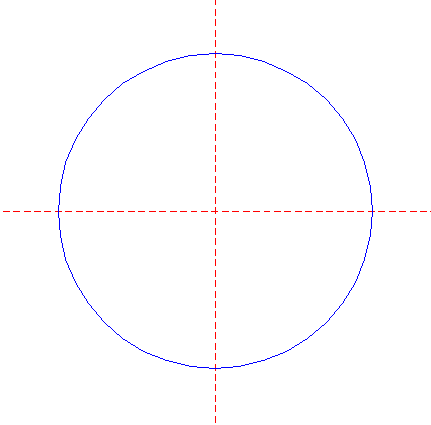Draw a circle. Draw and extend the horizontal and vertical centerlines. 2.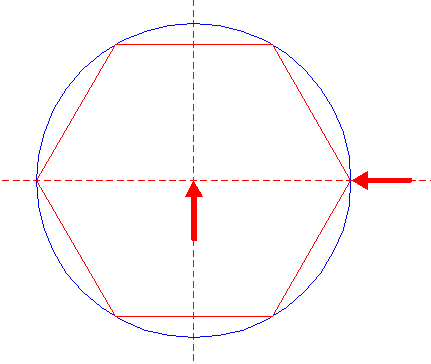Construct the inscribed hexagon (regular 6-sided polygon) of circle 1, pointing to the right. 3.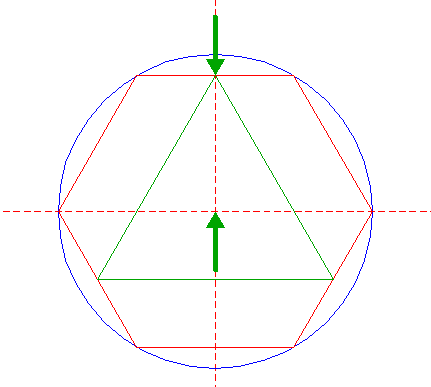Construct the inscribed equilateral triangle of hexagon 2, pointing up. 4.Construct the inscribed equilateral triangle of hexagon 2, pointing down. 5.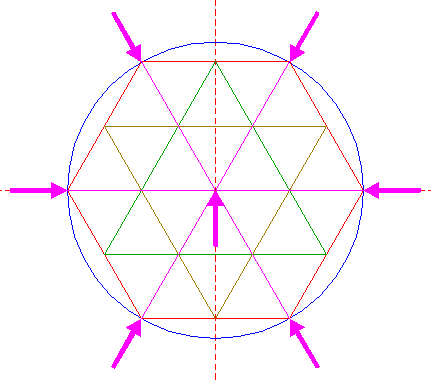Draw the six rays of hexagon 2, from the center to the angular points. 6.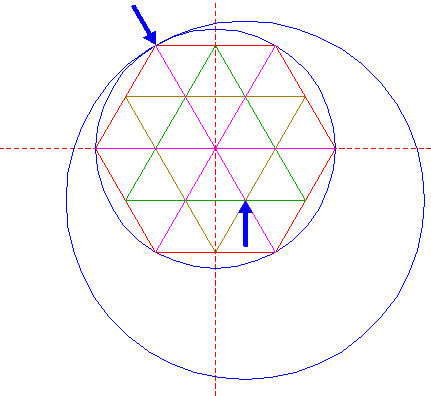Construct a circle centered at the lower righthand intersection of triangles 3 and 4, passing through the upper lefthand angular point of hexagon 2. 7.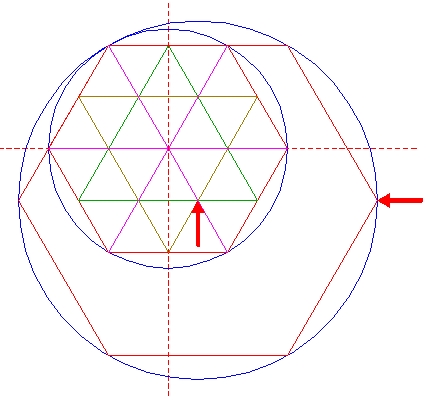Construct the inscribed hexagon of circle 6, pointing to the right. 8.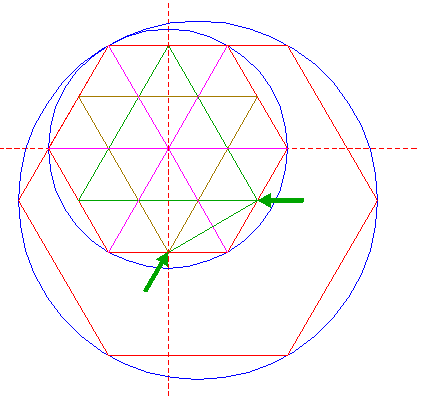Draw the connecting line between the lower righthand angular point of triangle 3 and the lower angular point of triangle 4. 9.Construct a circle centered at the intersection of line 8 and the lower righthand ray 5, passing through the center of circle 1. 10.Construct the circumscribed hexagon of circle 9, pointing to the right, and extend the lower righthand side in both directions upto hexagon 7. 11.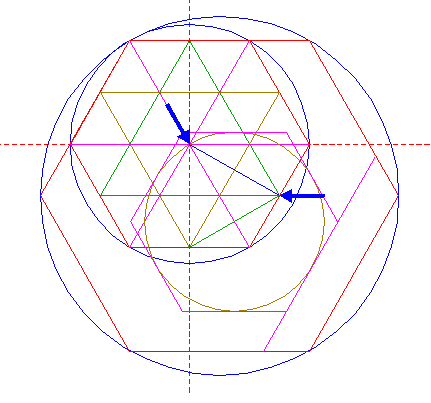Draw the connecting line between the center of circle 1 and the lower righthand angular point of triangle 3. 12.Construct a circle centered at the intersection of line 11 and the righthand side of triangle 4, passing through the lower angular point of this triangle. 13.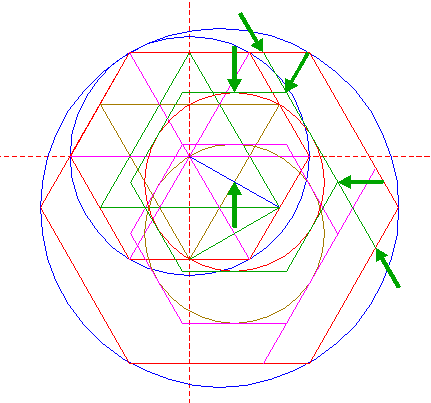Construct the circumscribed hexagon of circle 12, pointing to the right, and extend the upper righthand side in both directions upto hexagon 7. 14.Remove all parts from hexagon 7, and the extended sides of hexagons 10 and 13, ... 15.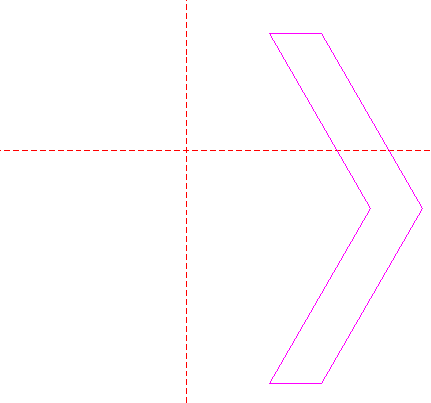...such that the shown pattern remains. 16.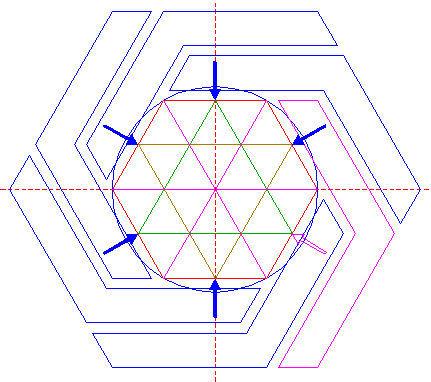Repeat all the necessary steps to copy pattern 15 five times, with respect to the other sides of hexagon 2. 17.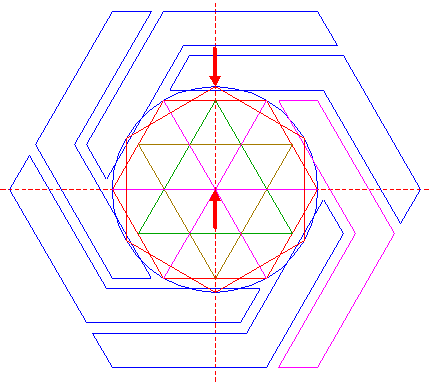Construct the inscribed hexagon of circle 1, pointing up. 18.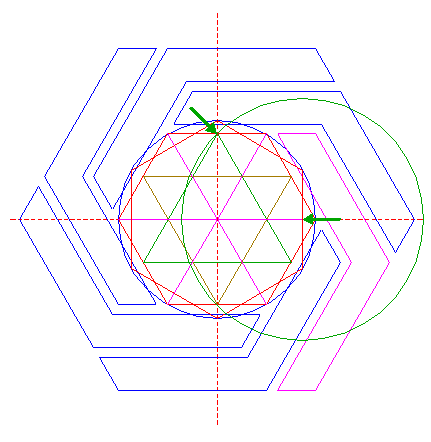Construct a circle centered at the intersection of the righthand side of hexagon 17 and the horizontal centerline, passing through the top of triangle 3. 19.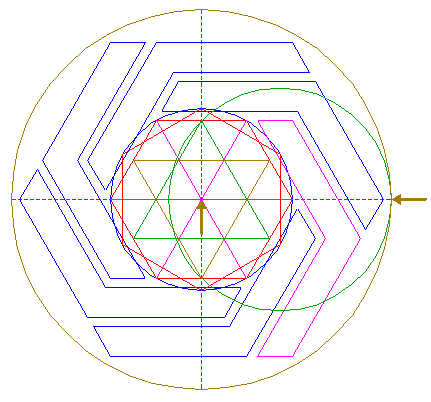Construct a circle concentric to circle 1, tangent to circle 18 at the righthand side. 20.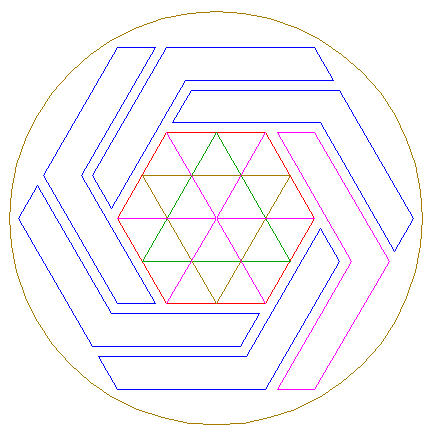Circle 19, hexagon 2, triangles 3 and 4, lines 5, and patterns 15 and 16, are used for the final reconstruction. 21.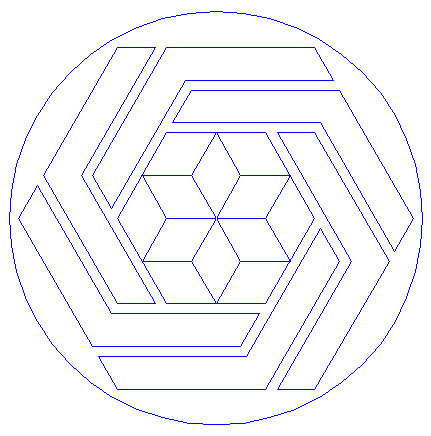Remove all parts not visible within the formation itself. 22.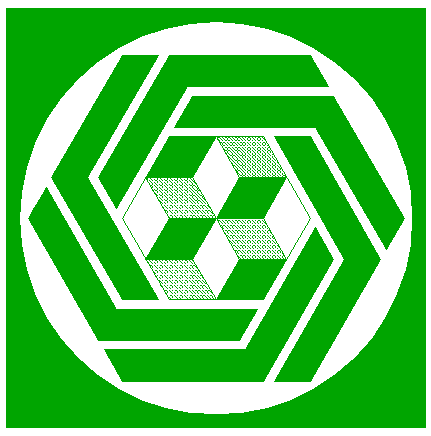Colour all areas corresponding to standing... 23.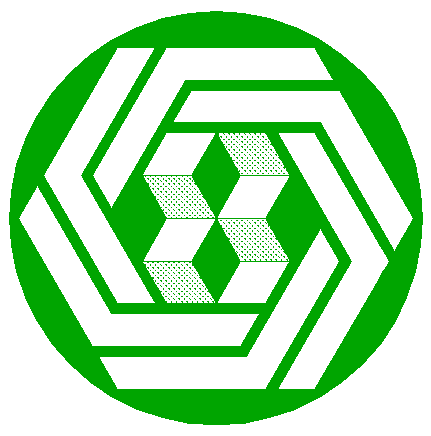...or to flattened crop, and finish the reconstruction of the 2020 Stanton St. Bernard formation. 24.The final result, matched with the aerial image.